# Servo Motor Control by Flex Sensor

Published  November 30, 2015   4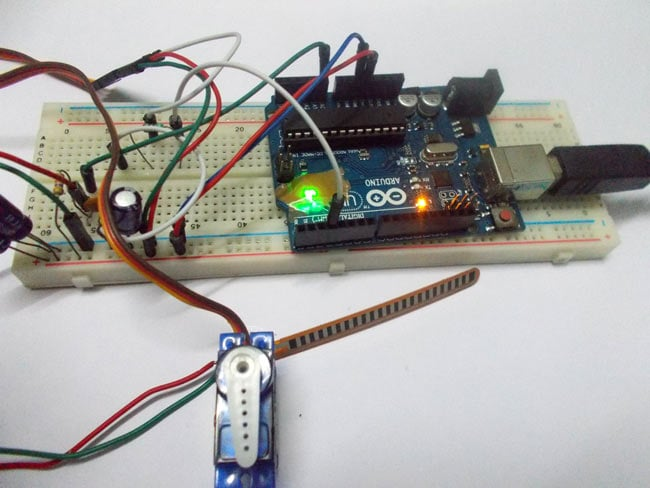In this tutorial we are going to develop a circuit using FLEX sensor, Arduino Uno and a Servo motor. This project is a servo control system where the servo shaft position is determined by the flex or bent or deviation of the FLEX sensor.

Lets first talk a bit about servo motors. Servo Motors are used where there is a need for accurate shaft movement or position. These are not proposed for high speed applications. These are proposed for low speed, medium torque and accurate position application. These motors are used in robotic arm machines, flight controls and control systems. Servo motors are used in embedded systems like vending machines etc.

Servo motors are available at different shapes and sizes. A servo motor will have mainly there wires, one is for positive voltage another is for ground and last one is for position setting. The RED wire is connected to power, Black wire is connected to ground and YELLOW wire is connected to signal.

A servo motor is a combination of DC motor, position control system, gears. The position of the shaft of the DC motor is adjusted by the control electronics in the servo, based on the duty ratio of the PWM signal the SIGNAL pin.

Simply speaking the control electronics adjust shaft position by controlling DC motor. This data regarding position of shaft is sent through the SIGNAL pin. The position data to the control should be sent in the form of PWM signal through the Signal pin of servo motor.

The frequency of PWM (Pulse Width Modulated) signal can vary based on type of servo motor. The important thing here is the DUTY RATIO of the PWM signal. Based on this DUTY RATION the control electronics adjust the shaft. For the shaft to be moved to 9o clock the TURN ON RATION must be 1/18.ie. 1 milli second of ‘ON time’ and 17 milli second of ‘OFF time’ in a 18 ms signal.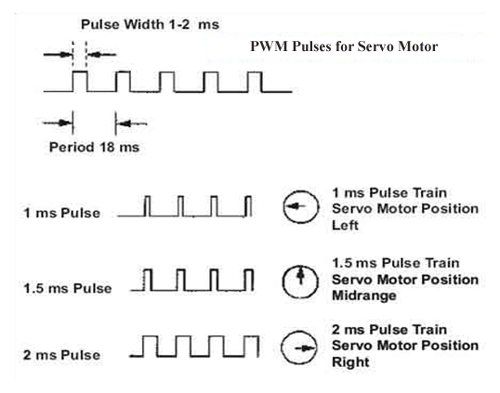For the shaft to be moved to 12o clock the ON time of signal must be 1.5ms and OFF time should be 16.5ms. This ratio is decoded by control system in servo and it adjusts the position based on it.

This PWM in here is generated by using ARDUINO UNO. So for now we know that, we can control the servo motor shaft by varying the duty ratio of PWM signal generated by Arduino Uno. The UNO has a special function which enables us to provide the position of SERVO without troubling the PWM signal. However it is important to know the PWM duty ration - servo position relation. We will talk more about it in description.

Now let’s talk about FLEX SENSOR. To interface a FLEX sensor to ARDUINO UNO,  we are going use 8 bit ADC (Analog to Digital Conversion) feature to do the job. A FLEX sensor is a transducer which changes its resistance when its shape is changed.  A FLEX sensor is of 2.2 inches long or of finger length. It is shown in figure.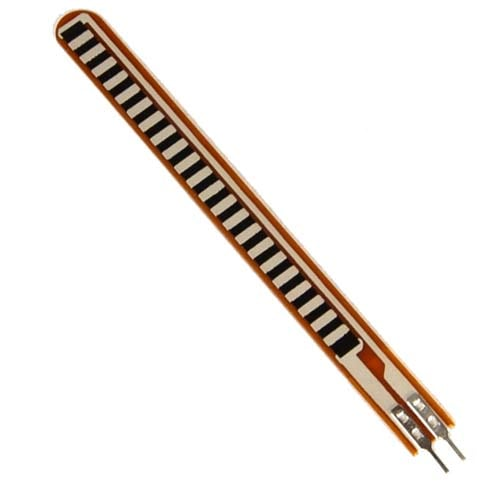Flex sensor is a transducer which changes its resistance when the linear surface is bent. Hence the name flex sensor. Simply speaking the sensor terminal resistance increases when it’s bent. This is shown in below figure.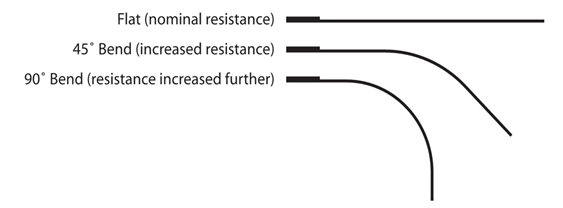This change in resistance can do no good unless we can read them. The controller at hand can only read the chances in voltage and nothing less, for this we are going to use voltage divider circuit, with that we can derive the resistance change as voltage change.

Voltage divider is a resistive circuit and is shown in figure. In this resistive network we have one constant resistance and other variable resistance. As shown in figure, R1 here is a constant resistance and R2 is FLEX sensor which acts as a resistance.

The midpoint of branch is taken to measurement. With R2 change, we have change at Vout. So with this we have a voltage which changes with weight.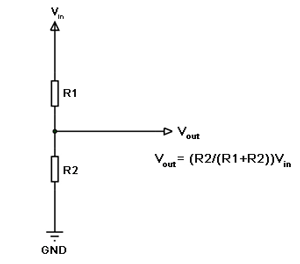Now important thing to note here is, the input taken by the controller for ADC conversion is as low as 50µAmp. This loading effect of resistance based voltage divider is important as the current drawn from Vout of voltage divider increases the error percentage increases, for now we need not worry about loading effect.

FLEX SENSOR when bent its resistance changes. With this transducer connected to a voltage divider circuit, we will have a changing voltage with FLEX on transducer. This variable voltage is FED to one of ADC channels, we will have a digital value relating to FLEX.

We will match this digital value to servo position, with this we will have servo control by flex.

### Components

Hardware: Arduino Uno, Power supply (5v), 1000 uF capacitor, 100nF capacitor (3 pieces), 100KΩ resistor, SERVO MOTOR (SG 90), 220Ω resistor, FLEX sensor.

Software: Atmel studio 6.2 or Aurdino nightly.

### Circuit Diagram and Explanation

The circuit diagram for servo motor control by FLEX sensor is shown in below figure.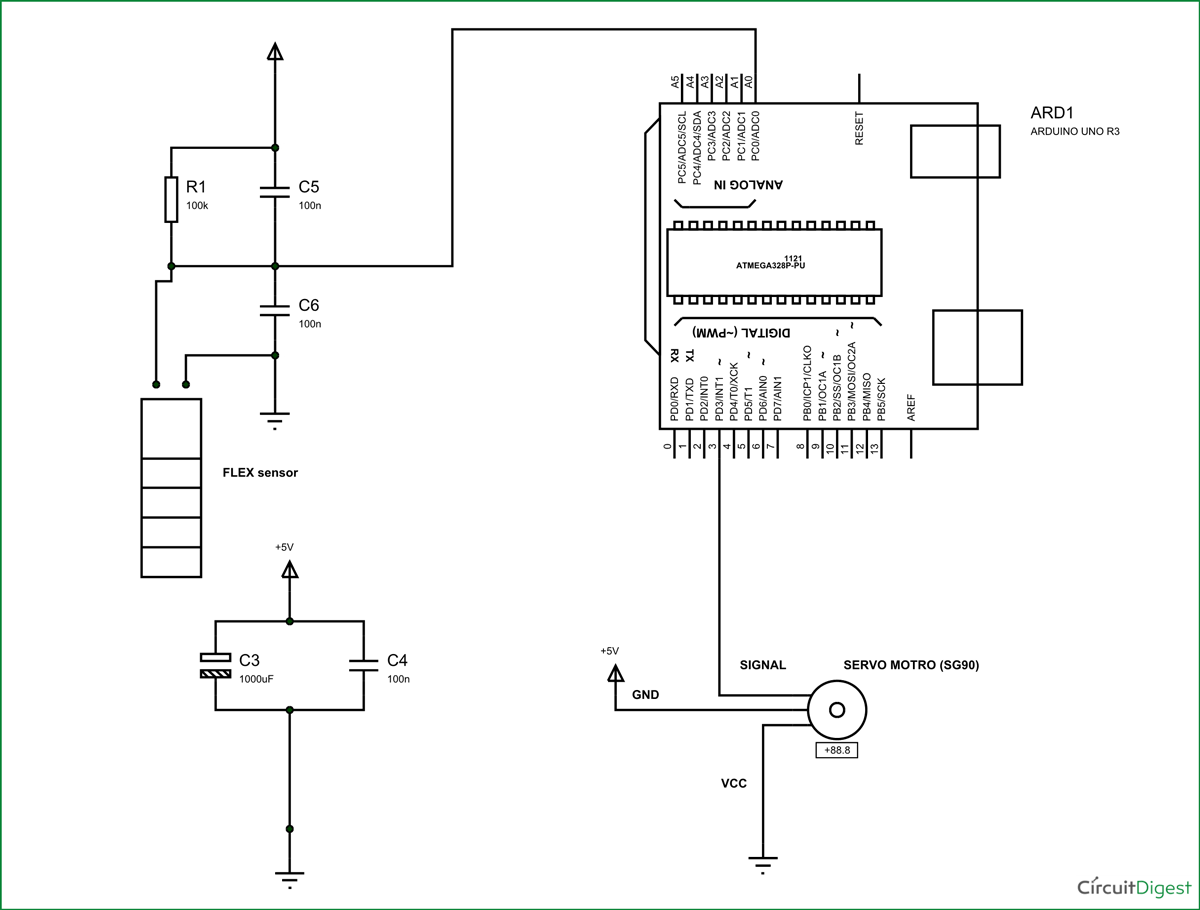The voltage across sensor is not completely linear; it will be a noisy one. To filter out the noise, capacitors are placed across each resistor in the divider circuit as shown in figure.

Here we are going to take the voltage provided by the divider (voltage which represents weight linearly) and feed it into one of ADC Channels of Arduino UNO. We are going to use A0 for this. After the ADC initialization, we will have digital value representing the bent on sensor. We will take this value and match it with servo position.

For this to happened we need to establish few instructions in program and we will talk about them in detail below.

ARDUINO has six ADC channels, as show in figure. In those any one or all of them can be used as inputs for analog voltage. The UNO ADC is of 10 bit resolution (so the integer values from (0-(2^10) 1023)).This means that it will map input voltages between 0 and 5 volts into integer values between 0 and 1023. So for every (5/1024= 4.9mV) per unit.

Here we are going to use A0 of UNO.

We need to know a few things.

First of all the UNO ADC channels has a default reference value of 5V. This means we can give a maximum input voltage of 5V for ADC conversion at any input channel. Since some sensors provide voltages from 0-2.5V, with a 5V reference we get lesser accuracy, so we have a instruction that enables us to change this reference value.  So for changing the reference value we have (“analogReference();”) For now we leave it as.

As default we get the maximum board ADC resolution which is 10bits, this resolution can be changed by using instruction (“analogReadResolution(bits);”). This resolution change can come in handy for some cases. For now we leave it as.

Now if the above conditions are set to default, the we can read value from ADC of channel ‘0’ by directly calling function “analogRead(pin);”, here “pin” represents pin where we connected analog signal, in this case it would be “A0”.

The value from ADC can be taken into an integer as “int SENSORVALUE = analogRead(A0); ”, by this instruction the value after ADC gets stored in the integer “SENSORVALUE”.

Now let’s talk about the SERVO, the UNO has a feature which enables us to control the servo position by just giving the degree value.  Say if we want the servo to be at 30, we can directly represent the value in the program. The SERVO header file takes care of all the duty ratio calculations internally.

 #include Servo servo; servo.attach(3); servo.write(degrees);

First statement represents the header file for controlling the SERVO MOTOR.

Second statement is naming the servo; we leave it as servo itself.

Third statement states where the servo signal pin is connected; this must be a PWM pin. Here we are using PIN3.

Fourth statement gives commands for positioning servo motor and is in degrees. If it is given 30, the servo motor rotates 30 degrees.

Now the sg90 can move from 0-180 degrees, we have ADC result 0-1024

So ADC is approximately six times the SERVO POSITION. So by divided the ADC result by 6 we will get the approximate SERVO hand position.

With this we will have servo position value fed to servo motor, which is in proportion to flex or bent. When this flex sensor mounted on glove, we can control servo position by movement of hand.

Code

#include <Servo.h>

Servo servo; //keeping name of servo SERVO itself

int sensorvalue =0;

void setup()

{

pinMode(A0,INPUT);// voltage divider value input

pinMode(3,OUTPUT);// PWM output to servo

servo.attach(3);// telling where signal pin of servo attached(must be a PWM pin)

}

void loop()

{

servo.write((sensorvalue-250)/2); //to avoid initial positioning of servo we need to neutralize the default voltage provided by voltage divider( setting servo position based on ADC result)

}

Video

Tags

Submitted by Gage on Thu, 11/03/2016 - 07:00

### Flex sensor and servo motor

Does the servo motor react when you bend the flex sensor in the opposite direction as seen in the video?

### The flex sensor is designed

The flex sensor is designed to be bent in just one direction and bending in other direction may damage the sensor and you will not get reliable data.

Submitted by jeyasuriya on Wed, 05/24/2017 - 13:05